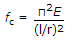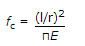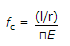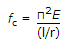# Civil Engineering - Steel Structure Design - Discussion

### Discussion :: Steel Structure Design - Section 1 (Q.No.7)

7.

The critical stress on a column for elastic buckling given by Euler's formula, is

 [A].[B].[C].[D].Explanation:

No answer description available for this question.

 Suresh said: (Jun 3, 2016) Give the expansion of each letter.

 Kutu said: (Jun 3, 2016) Critical stress = Criticl load/Area. Critical Load as per Euler formula = (pie sq x EI)/L2. So C.Strees = Pie Sq X E/ (L/r) Sq.

 Chhaya said: (Apr 28, 2017) Here R= √i/A. Radius of gyration.

 Hareesh said: (Nov 28, 2017) Radius of gyration r =π(I/A). I= A*r^2. Critical load P=(π^2*EI)/l^2. Both sides division with "A". P/A = (π^2*EAr^2)/(A*l^2). P/A = critical stress. Critical stress= (π^2*E)/(l/r)^2.